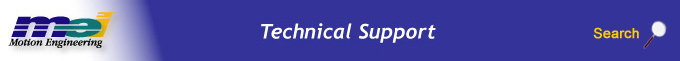.

MPIMotionPVTF

Definition

 ```typedef struct MPIMotionPVTF { long pointCount; double *position; double *velocity; double *feedforward; double *time; MPIMotionPoint point; } MPIMotionPVTF;```

Description

MPIMotionPVTF contains the motion parameters for the MPIMotionTypePVTF.

The feedforward values are interpolated linearly over the PT or PVT time intervals. The feedfoward values correspond to the P or PV values. (i.e. When the motion reaches a specified position (PTF) or position and velocity (PVTF), the interpolated feedforward value will be equal to what is specified in the motion parameters.)

The feedforward values are not set to zero at the beginning of the move; they retain the last value that is specified in the PTF or PVTF motion parameters.

The feedforward values are not zeroed by non-PTF or PVTF moves (i.e. PT, PVT, Spline, S-Curve, etc.).

 pointCount This value specifies the number of points. *position This array stores the positions for the motion profile. There is one position value per point, per axis. The length of the array must be equal to pointCount multiplied by the number of axes. The positions are interleaved in the array by the axis index. *velocity This array stores the velocities for the motion profile. There is one velocity value per point, per axis. The length of the array must be equal to pointCount multiplied by the number of axes. The velocities are interleaved in the array by the axis index. *feedforward This array stores the feedforward values for the motion profile. There is one feedforward value per point, per axis. The length of the array must be equal to pointCount multiplied by the number of axes. The feedforward values are interleaved in the array by the axis index. The units are raw DAC counts (range -32768 to +32767). *time This array stores the times for the motion profile. There is one time value per point. The time specifies the number of seconds between the specified position, and the previous position (point). The length of the time array must be equal to pointCount. point This structure contains the point configuration. Please see MPIMotionPoint data type for more information.